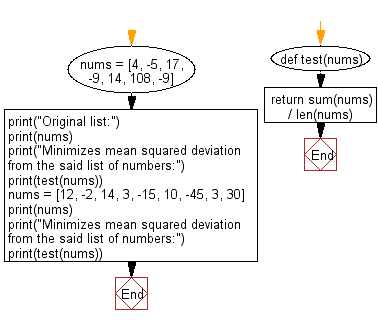﻿ Python: Find x that minimizes mean squared deviation from a given list of numbers - w3resource# Python: Find x that minimizes mean squared deviation from a given list of numbers

## Python Programming Puzzles: Exercise-27 with Solution

Squared deviations from the mean (SDM) are involved in various calculations. In probability theory and statistics, the definition of variance is either the expected value of the SDM (when considering a theoretical distribution) or its average value (for actual experimental data). Computations for analysis of variance involve the partitioning of a sum of SDM.
Write a Python program to find x that minimizes mean squared deviation from a given a list of numbers.
The problem requires minimizing the sum of squared deviations, which turns out to be the mean mu. Moreover, if mu is the mean of the numbers then a simple calculation.

```Input:
[4, -5, 17, -9, 14, 108, -9]
Output:
17.142857142857142
Input:
[12, -2, 14, 3, -15, 10, -45, 3, 30]
Output:
1.1111111111111112
```

Sample Solution:

Python Code:

``````#License: https://bit.ly/3oLErEI

def test(nums):
return sum(nums) / len(nums)
nums = [4, -5, 17, -9, 14, 108, -9]
print("Original list:")
print(nums)
print("Minimizes mean squared deviation from the said list of numbers:")
print(test(nums))
nums = [12, -2, 14, 3, -15, 10, -45, 3, 30]
print("Original list:")
print(nums)
print("Minimizes mean squared deviation from the said list of numbers:")
print(test(nums))
``````

Sample Output:

```Original list:
[4, -5, 17, -9, 14, 108, -9]
Minimizes mean squared deviation from the said list of numbers:
17.142857142857142
Original list:
[12, -2, 14, 3, -15, 10, -45, 3, 30]
Minimizes mean squared deviation from the said list of numbers:
1.1111111111111112
```

Flowchart:## Visualize Python code execution:

The following tool visualize what the computer is doing step-by-step as it executes the said program:

Python Code Editor :

Have another way to solve this solution? Contribute your code (and comments) through Disqus.

What is the difficulty level of this exercise?

Test your Programming skills with w3resource's quiz.

﻿

## Python: Tips of the Day

Clamps num within the inclusive range specified by the boundary values x and y:

Example:

```def tips_clamp_num(num,x,y):
return max(min(num, max(x, y)), min(x, y))
print(tips_clamp_num(2, 4, 6))
print(tips_clamp_num(1, -1, -6))
```

Output:

```4
-1
```